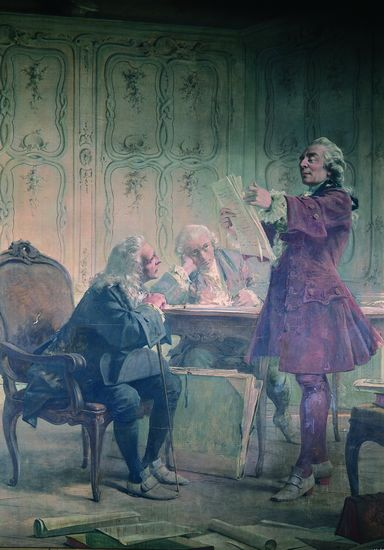## Buffon machinesBy chance I came across a 2010 paper entitled On Buffon Machines and Numbers by Philippe Flajolet, Maryse Pelletier and Michèle Soria. Which relates to Bernoulli factories, a related riddle, and the recent paper by Luis Mendo I reviewed here. What the authors call a Buffon machine is a device that produces a perfect simulation of a discrete random variable out of a uniform bit generator. Just like (George Louis Leclerc, comte de) Buffon’s needle produces a Bernoulli outcome with success probability π/4. out of a real Uniform over (0,1). Turned into a sequence of Uniform random bits.

“Machines that always halt can only produce Bernoulli distributions whose parameter is a dyadic rational.”

When I first read this sentence it seemed to clash with the earlier riddle, until I realised the later started from a B(p) coin to produce a fair coin, while this paper starts with a fair coin.

The paper hence aims at a version of the Bernoulli factory problem (see Definition 2), although the term is only mentioned at the very end, with the added requirement of simplicity and conciseness translated as a finite expected number of draws and possibly an exponential tail.

It first recalls the (Forsythe–)von Neumann method of generating exponential (and other) variates out of a Uniform generator (see Section IV.2 in Devroye’s generation bible). Expanded into a general algorithm for generating discrete random variables whose pmf’s are related to permutation cardinals,$\mathbb P(N=n)\propto P_n\lambda^n/n!$

if the Bernoulli generator has probability λ. These include the Poisson and the logarithmic distributions and as a side product Bernoulli distributions with some logarithmic, exponential and trigonometric transforms of λ.

As a side remark, a Bernoulli generator with probability 1/π is derived from Ramanujan identity$\frac{1}{\pi} = \sum_{n=0}^\infty {2n \choose n}^3 \frac{6n+1}{2^{8n+2}}$

as “a discrete analogue of Buffon’s original. In a neat connection with Mendo’s paper, the authors of this 2010 paper note that Euler’s constant does not appear to be achievable by a Buffon machine.

### 2 Responses to “Buffon machines”

1.xi'an Says:

Thanks for pointing out the mistake, Peter.

2.Peter Occil Says:

Luis Mendo’s article started when I let him know of a question that is also found in the Buffon machines paper, on whether a “natural” algorithm that exactly simulates Euler’s constant using only random bits exists. Also, the identity for 1/pi given in the Buffon machines paper (and in this blog post) is unfortunately incorrect (although the algorithm isn’t): instead of “8n+4” or “8+4”, read “8n+2”. I found this error while writing a [derivation of the algorithm](https://peteroupc.github.io/bernoulli.html#Sketch_of_Derivation_of_the_Algorithm_for_1___pi), and [C.F.F. Karney independently did too](http://randomlib.sourceforge.net/html/classRandomLib_1_1InversePiProb.html).

This site uses Akismet to reduce spam. Learn how your comment data is processed.